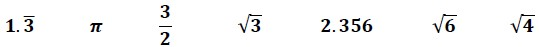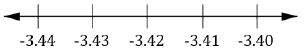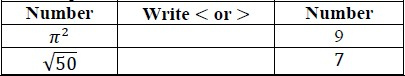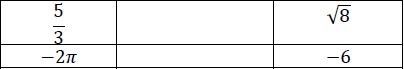# Standard #: MA.8.NSO.1.2

This document was generated on CPALMS - www.cpalms.org

Plot, order and compare rational and irrational numbers, represented in various forms.

### Clarifications

Clarification 1: Within this benchmark, it is not the expectation to work with the number e.

Clarification 2: Within this benchmark, the expectation is to plot, order and compare square roots and cube roots.

Clarification 3: Within this benchmark, the expectation is to use symbols (<, > or =).

### General Information

Subject Area: Mathematics (B.E.S.T.)
Strand: Number Sense and Operations
Status: State Board Approved

### Terms from the K-12 Glossary

• Irrational Numbers
• Rational Numbers

### Vertical Alignment

Previous Benchmarks

Next Benchmarks

### Purpose and Instructional Strategies

In grade 7, students expressed rational numbers with terminating and repeating decimals. In grade 8, students define irrational numbers, recognizing and expressing them in various forms, and students compare rational numbers to irrational numbers. In Algebra 1, students will perform operations with radicals. In Geometry, students will extend their understanding of radical approximations to weighted averages on a number line.
• Students should have the opportunity to draw number lines with appropriate scales to plot the numbers to provide an understanding of where the numbers are in relation to numbers that are greater than and less than the number to be plotted.
• Students locate and compare rational and irrational numbers on the number line. Additionally, students understand that the value of a square root or a cube root can be approximated between integers.
• For example, to find an approximation of $\sqrt{28}$, two methods are described below, each using the nearest perfect squares to the radicand:
• determine the perfect squares 28 is between, which would be 25 and 36. The square roots of 25 and 36 are 5 and 6, respectively, so we know that $\sqrt{28}$ is between 5 and 6. Since 28 is closer to 25, an estimate of the square root would be closer to 5.
• since 28 is located $\frac{\text{3}}{\text{11}}$ of the distance from 25 to 36, the $\sqrt{28}$ is approximately located 11 of the distance from $\sqrt{25}$ to $\sqrt{36}$. So, this reasoning gives the approximation $\sqrt{25}$ + $\frac{\text{3}}{\text{11}}$, which is about 5.27. This method particularly relevant when students determine weighted averages on a number line in Geometry.
• Students also recognize that every positive number has both a positive and a negative square root. The negative square root of $n$ is written as −$\sqrt{n}$.
• Instruction includes the use of technology, including a calculator.

### Common Misconceptions or Errors

• Students may not understand that square and cube roots can be plotted on a number line.

### Strategies to Support Tiered Instruction

• Instruction includes providing students with examples of square and cube roots for them to place on a number line and facilitating a conversation on understanding the value of each square and cube root.
• Teacher provides opportunities to co-construct number lines with appropriate scales and plot approximate values of cube roots and square roots.
• For example, provide partially completed examples of non-perfect square roots and non-perfect cube roots by using perfect square roots and perfect cube roots as benchmark quantities.
• Teacher provides support in recognizing that every positive number has both a positive and negative square root.
• For example, show examples of how multiplying two negative numbers gives a positive number, and how the square root of a number can be both positive and negative.
• Teacher assists students in writing an inequality to represent written statements.
• For example:
• Monique has more books than Mary.
• Mark has 5 pencils and Barry has 8.
• Animal Kingdom has at least 100 different species of animals.

Below are irrational and rational numbers.• Part A. Order the numbers from least to greatest by plotting on a number line.
• Part B. Identify which numbers are irrational.
• Part C. Write an inequality that compares a rational number and an irrational number from the list.

### Instructional Items

Instructional Item 1
Plot −3.42857… on the number line below and explain how you determined its location.Instructional Item 2
Using the chart below, compare the irrational and rational numbers shown.Instructional Item 3
Plot the following cube roots on a number line $\sqrt{8}$, $\sqrt{10}$ and $\sqrt{27}$.

*The strategies, tasks and items included in the B1G-M are examples and should not be considered comprehensive.

#### Related Courses

 Course Number1111 Course Title222 1200400: Foundational Skills in Mathematics 9-12 (Specifically in versions: 2014 - 2015, 2015 - 2022, 2022 and beyond (current)) 1205050: M/J Accelerated Mathematics Grade 7 (Specifically in versions: 2014 - 2015, 2015 - 2020, 2020 - 2022, 2022 and beyond (current)) 1205070: M/J Grade 8 Pre-Algebra (Specifically in versions: 2014 - 2015, 2015 - 2022, 2022 and beyond (current)) 1204000: M/J Foundational Skills in Mathematics 6-8 (Specifically in versions: 2014 - 2015, 2015 - 2022, 2022 and beyond (current)) 7812030: Access M/J Grade 8 Pre-Algebra (Specifically in versions: 2014 - 2015, 2015 - 2018, 2018 - 2019, 2019 - 2022, 2022 and beyond (current))

#### Related Access Points

 Access Point Number Access Point Title MA.8.NSO.1.AP.2 Use appropriate tools to plot, order, and compare simple square roots and cube roots for quantities less than 100.

#### Formative Assessments

 Name Description The Irrational Beauty of the Golden Ratio Students are asked to find and interpret lower and upper bounds of an irrational expression using a calculator. Approximating Irrational Numbers Students are asked to plot the square root of eight on three number lines, scaled to progressively more precision. Locating Irrational Numbers Students are asked to graph three different irrational numbers on number lines. Comparing Irrational Numbers Students are asked to estimate the value of several irrational numbers using a calculator and order them on a number line.
Printed On:9/28/2023 2:11:39 AM
Print Page | Close this window